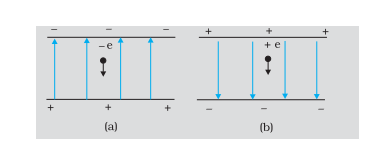Home/Class 12/Physics/

An electron falls through a distance of  $$1.5\;\text{cm}$$  in a uniform electric field of magnitude $$2.0\times 10^4NC^{-1}.$$  The direction of the field is reversed keeping its magnitude unchanged and a proton falls through the same distance $$.$$ Compute the time of fall in each case. Contrast the situation with that of ‘free fall under gravity’.Speed
00:00
07:31## QuestionPhysicsClass 12

An electron falls through a distance of  $$1.5\;\text{cm}$$  in a uniform electric field of magnitude $$2.0\times 10^4NC^{-1}.$$  The direction of the field is reversed keeping its magnitude unchanged and a proton falls through the same distance $$.$$ Compute the time of fall in each case. Contrast the situation with that of ‘free fall under gravity’.See the analysis below
4.64.6## Solution

Let us consider the magnitude of the electric field be $$\mathit{E.}$$
In the field shown is upward, hence the negatively charged electrons experience a downward force of magnitude  $$\mathit{eE.}$$
i.e.,  $$F=\mathit{eE}$$
The acceleration of the electron is
$$a_e=\frac{\mathit{eE}}{m_e}$$
where  $$m_e$$  is the mass of the electron.
Let us consider the falling distance be  $$\text{ h }.$$
From rest, the time required by the electron to fall through a distance  $$h$$  is given as,
$$t_e=\sqrt{\frac{2h}{a_e}}$$  $$=\sqrt{\frac{2\mathit{hm}_e}{\mathit{eE}}}$$
For,  $$e=1.6\times 10^{-19}C,m_e=9.11\times 10^{-31}\mathit{kg}$$
$$E=2.0\times 10^4NC^{-1},h=1.5\times 10^{-2}m$$
The time of fall for the electron can be given as,  $$t_e=2.9\times 10^{-9}s$$
In the field is shown downward, and hence the positively charged proton experiences a downward force of magnitude  $$\mathit{eE.}$$
The acceleration of the proton is
$$a_p=\frac{\mathit{eE}}{m_p}$$
where  $$m_p=1.67\times 10^{-27}\mathit{kg}$$  is the mass of the proton.
Then the time of fall for the proton can be given as,
$$t_p=\sqrt{\frac{2h}{a_p}}$$
$$=\sqrt{\frac{2\mathit{hm}_p}{\mathit{eE}}}$$
$$t_p=1.3\times 10^{-7}s$$
This shows that the heavier particle proton takes a greater time to fall through the same distance as electron do. This is contrast to the situation of ‘free fall under gravity’ where the time of fall is independent to the mass of the body. But on solving for time of fall, we neglected the acceleration due to gravity.
To justify this, we shall compute for acceleration of the proton in the given electric field as,
$$a_p=\frac{\mathit{eE}}{m_p}=\frac{(1.6\times 10)^{-19})\times (2\times 10^4)}{1.67 \times 10^{-27}}$$
$$a_p={1.9 \times 10^{12}}\;m/s^2$$
which is very much greater as compared to the value of acceleration due to gravity $$( g =9.8\; m/s^2).$$ The acceleration of the electron is even more greater. So, the effect of acceleration due to gravity can be neglected here.